### PROC OPTLP Statement

PROC OPTLP <options> ;

You can specify the following options in the PROC OPTLP statement.

#### Data Set Options

DATA=SAS-data-set

specifies the input data set corresponding to the LP model. If this option is not specified, PROC OPTLP will use the most recently created SAS data set. See Chapter 15: The MPS-Format SAS Data Set, for more details about the input data set.

DUALIN=SAS-data-set
DIN=SAS-data-set

specifies the input data set corresponding to the dual solution that is required for warm starting the primal and dual simplex solvers. See the section Data Input and Output for details.

DUALOUT=SAS-data-set
DOUT=SAS-data-set

specifies the output data set for the dual solution. This data set contains the dual solution information. See the section Data Input and Output for details.

OBJSENSE=option

specifies whether the LP model is a minimization or a maximization problem. You specify OBJSENSE=MIN for a minimization problem and OBJSENSE=MAX for a maximization problem. Alternatively, you can specify the objective sense in the input data set; see the section ROWS Section for details. If for some reason the objective sense is specified differently in these two places, this option supersedes the objective sense specified in the input data set. If the objective sense is not specified anywhere, then PROC OPTLP interprets and solves the linear program as a minimization problem.

PRIMALIN=SAS-data-set
PIN=SAS-data-set

specifies the input data set corresponding to the primal solution that is required for warm starting the primal and dual simplex solvers. See the section Data Input and Output for details.

PRIMALOUT=SAS-data-set
POUT=SAS-data-set

specifies the output data set for the primal solution. This data set contains the primal solution information. See the section Data Input and Output for details.

SAVE_ONLY_IF_OPTIMAL

specifies that the PRIMALOUT= and DUALOUT= data sets be saved only if the final solution obtained by the solver at termination is optimal. If the PRIMALOUT= and DUALOUT= options are specified, then by default (that is, omitting the SAVE_ONLY_IF_OPTIMAL option), PROC OPTLP always saves the solutions obtained at termination, regardless of the final status. If the SAVE_ONLY_IF_OPTIMAL option is not specified, the output data sets can contain an intermediate solution, if one is available.

#### Solver Options

IIS=number | string

specifies whether PROC OPTLP attempts to identify a set of constraints and variables that form an irreducible infeasible set (IIS). Table 10.2 describes the valid values of the IIS= option.

Table 10.2: Values for IIS= Option

number

string

Description

0

OFF

Disables IIS detection.

1

ON

Enables IIS detection.

If an IIS is found, information about infeasible constraints or variable bounds can be found in the DUALOUT= and PRIMALOUT= data sets. The default value of this option is OFF. See the section Irreducible Infeasible Set for details.

ALGORITHM=option
SOLVER=option
SOL=option

specifies one of the following LP solvers:

Option

Description

PRIMAL (PS)

Uses primal simplex solver.

DUAL (DS)

Uses dual simplex solver.

NETWORK (NS)

Uses network simplex solver.

INTERIORPOINT (IP)

Uses interior point solver.

CONCURRENT (CON) (experimental)

Uses several different algorithms in parallel.

The valid abbreviated value for each option is indicated in parentheses. By default, the dual simplex solver is used.

ALGORITHM2=option
SOLVER2=option

specifies one of the following LP solvers if ALGORITHM=NS:

Option

Description

PRIMAL (PS)

Uses primal simplex solver (after network simplex).

DUAL (DS)

Uses dual simplex solver (after network simplex).

The valid abbreviated value for each option is indicated in parentheses. By default, the OPTLP procedure decides which algorithm is best to use after calling the network simplex solver on the extracted network.

#### Presolve Options

PRESOLVER=number | string
PRESOL=number | string

specifies one of the following presolve options:

number

string

Description

0

NONE

Disables presolver.

–1

AUTOMATIC

Applies presolver by using default setting.

1

BASIC

Performs basic presolve like removing empty rows, columns, and fixed variables.

2

MODERATE

Performs basic presolve and apply other inexpensive presolve techniques.

3

AGGRESSIVE

Performs moderate presolve and apply other aggressive (but expensive) presolve techniques.

The default option is AUTOMATIC (–1). See the section Presolve for details.

#### Control Options

FEASTOL=specifies the feasibility tolerance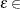[1E–9, 1E–4] for determining the feasibility of a variable value. The default value is 1E–6.

LOGFREQ=PRINTFREQ=specifies that the printing of the solution progress to the iteration log is to occur after everyiterations. The print frequency,, is an integer between zero and the largest four-byte signed integer, which is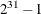.

The value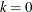disables the printing of the progress of the solution.

If the LOGFREQ= option is not specified, then PROC OPTLP displays the iteration log with a dynamic frequency according to the problem size if the primal or dual simplex solver is used, with frequency 10,000 if the network simplex solver is used, or with frequency 1 if the interior point solver is used.

LOGLEVEL=number | string
PRINTLEVEL2=number | string

controls the amount of information displayed in the SAS log by the LP solver, from a short description of presolve information and summary to details at each iteration. Table 10.3 describes the valid values for this option.

Table 10.3: Values for LOGLEVEL= Option

number

string

Description

0

NONE

Turn off all solver-related messages in SAS log.

1

BASIC

Display a solver summary after stopping.

2

MODERATE

Print a solver summary and an iteration log by using the interval dictated by the LOGFREQ= option.

3

AGGRESSIVE

Print a detailed solver summary and an iteration log by using the interval dictated by the LOGFREQ= option.

The default value is MODERATE.

MAXITER=specifies the maximum number of iterations. The valuecan be any integer between one and the largest four-byte signed integer, which is. If you do not specify this option, the procedure does not stop based on the number of iterations performed. For network simplex, this iteration limit corresponds to the solver called after network simplex (either primal or dual simplex).

MAXTIME=specifies an upper limit ofseconds of time for reading in the data and performing the optimization process. The value of the TIMETYPE= option determines the type of units used. If you do not specify this option, the procedure does not stop based on the amount of time elapsed. The value of t can be any positive number; the default value is the positive number that has the largest absolute value that can be represented in your operating environment.

OPTTOL=specifies the optimality tolerance[1E–9, 1E–4] for declaring optimality. The default value is 1E–6.

PRINTLEVEL=012

specifies whether a summary of the problem and solution should be printed. If PRINTLEVEL=1, then the ODS (Output Delivery System) tables ProblemSummary, SolutionSummary, and PerformanceInfo are produced and printed. If PRINTLEVEL=2, then these tables are produced and printed along with an additional table called ProblemStatistics. If PRINTLEVEL=0, then no ODS tables are produced or printed. The default value is 1.

For details about the ODS tables created by PROC OPTLP, see the section ODS Tables.

TIMETYPE=number | string

specifies whether CPU time or real time is used for the MAXTIME= option and the _OROPTLP_ macro variable in a PROC OPTLP call. Table 10.4 describes the valid values of the TIMETYPE= option.

Table 10.4: Values for TIMETYPE= Option

number

string

Description

0

CPU

Specifies units of CPU time.

1

REAL

Specifies units of real time.

The default value of the TIMETYPE= option depends on the values of the NTHREADS= and NODES= options in the PERFORMANCE statement. See the section PERFORMANCE Statement in Chapter 4: Shared Concepts and Topics. for more information about the NTHREADS= and NODES= options.

If you specify a value greater than 1 for either the NTHREADS= or the NODES= option, the default value of the TIMETYPE= option is REAL. If you specify a value of 1 for both the NTHREADS= and NODES= options, the default value of the TIMETYPE= option is CPU.

#### Simplex Algorithm Options

BASIS=number | string

specifies the following options for generating an initial basis:

number

string

Description

0

CRASH

Generate an initial basis by using crash techniques (Maros, 2003). The procedure creates a triangular basic matrix consisting of both decision variables and slack variables.

1

SLACK

Generate an initial basis by using all slack variables.

2

WARMSTART

Start the primal and dual simplex solvers with a user-specified initial basis. The PRIMALIN= and DUALIN= data sets are required to specify an initial basis.

The default option for the primal simplex solver is CRASH (0). The default option for the dual simplex solver is SLACK(1). For network simplex, this option has no effect.

PRICETYPE=number | string

specifies one of the following pricing strategies for the primal and dual simplex solvers:

number

string

Description

0

HYBRID

Use a hybrid of Devex and steepest-edge pricing strategies. Available for the primal simplex solver only.

1

PARTIAL

Use Dantzig’s rule on a queue of decision variables. Optionally, you can specify QUEUESIZE=. Available for the primal simplex solver only.

2

FULL

Use Dantzig’s rule on all decision variables.

3

DEVEX

Use Devex pricing strategy.

4

STEEPESTEDGE

Use steepest-edge pricing strategy.

The default pricing strategy for the primal simplex solver is HYBRID (0) and for the dual simplex solver is STEEPESTEDGE (4). For the network simplex solver, this option applies only to the solver specified by the ALGORITHM2= option. See the section Pricing Strategies for the Primal and Dual Simplex Solvers for details.

QUEUESIZE=specifies the queue size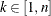, whereis the number of decision variables. This queue is used for finding an entering variable in the simplex iteration. The default value is chosen adaptively based on the number of decision variables. This option is used only when PRICETYPE=PARTIAL.

SCALE=number | string

specifies one of the following scaling options:

number

string

Description

0

NONE

Disable scaling.

–1

AUTOMATIC

Automatically apply scaling procedure if necessary.

The default option is AUTOMATIC (–1).

#### Interior Point Algorithm Options

CROSSOVER=number | string

specifies whether to convert the interior point solution to a basic simplex solution. If the interior point algorithm terminates with a solution, the crossover algorithm uses the interior point solution to create an initial basic solution. After performing primal fixing and dual fixing, the crossover algorithm calls a simplex algorithm to locate an optimal basic solution.

number

string

Description

0

OFF

Do not convert the interior point solution to a basic simplex solution.

1

ON

Convert the interior point solution to a basic simplex solution.

The default value of the CROSSOVER= option is OFF.

STOP_DG=
specifies the desired relative duality gap
[1E–9, 1E–4]. This is the relative difference between the primal and dual objective function values and is the primary solution quality parameter. The default value is 1E–6. See the section The Interior Point Algorithm for details.

STOP_DI=specifies the maximum allowed relative dual constraints violation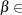[1E–9, 1E–4]. The default value is 1E–6. See the section The Interior Point Algorithm for details.

STOP_PI=specifies the maximum allowed relative bound and primal constraints violation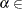[1E–9, 1E–4]. The default value is 1E–6. See the section The Interior Point Algorithm for details.